# Differentially flat systems¶

The control.flatsys package contains a set of classes and functions that can be used to compute trajectories for differentially flat systems.

A differentially flat system is defined by creating an object using the FlatSystem class, which has member functions for mapping the system state and input into and out of flat coordinates. The point_to_point() function can be used to create a trajectory between two endpoints, written in terms of a set of basis functions defined using the BasisFamily class. The resulting trajectory is return as a SystemTrajectory object and can be evaluated using the eval() member function. Alternatively, the solve_flat_ocp() function can be used to solve an optimal control problem with trajectory and final costs or constraints.

The docstring examples assume that the following import commands:

>>> import numpy as np
>>> import control as ct
>>> import control.flatsys as fs


## Overview of differential flatness¶

A nonlinear differential equation of the form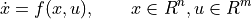is differentially flat if there exists a function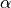such that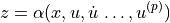and we can write the solutions of the nonlinear system as functions of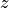and a finite number of derivatives

(1)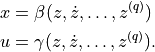For a differentially flat system, all of the feasible trajectories for the system can be written as functions of a flat output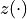and its derivatives. The number of flat outputs is always equal to the number of system inputs.

Differentially flat systems are useful in situations where explicit trajectory generation is required. Since the behavior of a flat system is determined by the flat outputs, we can plan trajectories in output space, and then map these to appropriate inputs. Suppose we wish to generate a feasible trajectory for the the nonlinear system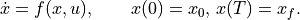If the system is differentially flat then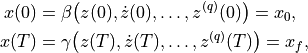and we see that the initial and final condition in the full state space depends on just the outputand its derivatives at the initial and final times. Thus any trajectory forthat satisfies these boundary conditions will be a feasible trajectory for the system, using equation (1) to determine the full state space and input trajectories.

In particular, given initial and final conditions onand its derivatives that satisfy the initial and final conditions any curvesatisfying those conditions will correspond to a feasible trajectory of the system. We can parameterize the flat output trajectory using a set of smooth basis functions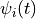: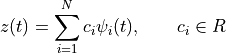We seek a set of coefficients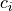,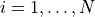such that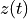satisfies the boundary conditions for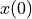and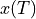. The derivatives of the flat output can be computed in terms of the derivatives of the basis functions: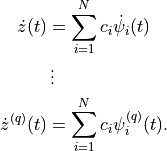We can thus write the conditions on the flat outputs and their derivatives as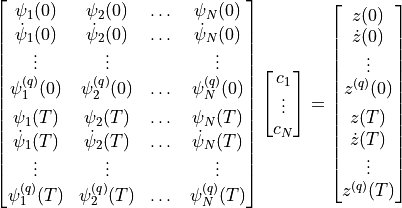This equation is a linear equation of the form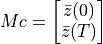where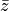is called the flat flag for the system. Assuming that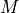has a sufficient number of columns and that it is full column rank, we can solve for a (possibly non-unique)that solves the trajectory generation problem.

## Module usage¶

To create a trajectory for a differentially flat system, a FlatSystem object must be created. This is done by specifying the forward and reverse mappings between the system state/input and the differentially flat outputs and their derivatives (“flat flag”).

The forward() method computes the flat flag given a state and input:

zflag = sys.forward(x, u)

The reverse() method computes the state and input given the flat flag:

x, u = sys.reverse(zflag)

The flagis implemented as a list of flat outputs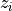and their derivatives up to order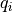:

zflag[i][j] =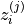The number of flat outputs must match the number of system inputs.

For a linear system, a flat system representation can be generated using the LinearFlatSystem class:

sys = control.flatsys.LinearFlatSystem(linsys)


For more general systems, the FlatSystem object must be created manually:

sys = control.flatsys.FlatSystem(
forward, reverse, states=['x1', ..., 'xn'], inputs=['u1', ..., 'um'])


In addition to the flat system description, a set of basis functions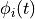must be chosen. The FlatBasis class is used to represent the basis functions. A polynomial basis function of the form 1,,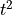, … can be computed using the PolyFamily class, which is initialized by passing the desired order of the polynomial basis set:

basis = control.flatsys.PolyFamily(N)


Additional basis function families include Bezier curves (BezierFamily) and B-splines (BSplineFamily).

Once the system and basis function have been defined, the point_to_point() function can be used to compute a trajectory between initial and final states and inputs:

traj = control.flatsys.point_to_point(
sys, Tf, x0, u0, xf, uf, basis=basis)


The returned object has class SystemTrajectory and can be used to compute the state and input trajectory between the initial and final condition:

xd, ud = traj.eval(T)


where T is a list of times on which the trajectory should be evaluated (e.g., T = numpy.linspace(0, Tf, M).

The point_to_point() function also allows the specification of a cost function and/or constraints, in the same format as solve_ocp().

The solve_flat_ocp() function can be used to solve an optimal control problem without a final state:

traj = control.flatsys.solve_flat_ocp(
sys, timepts, x0, u0, cost, basis=basis)


The cost parameter is a function function with call signature cost(x, u) and should return the (incremental) cost at the given state, and input. It will be evaluated at each point in the timepts vector. The terminal_cost parameter can be used to specify a cost function for the final point in the trajectory.

## Example¶

To illustrate how we can use a two degree-of-freedom design to improve the performance of the system, consider the problem of steering a car to change lanes on a road. We use the non-normalized form of the dynamics, which are derived Feedback Systems by Astrom and Murray, Example 3.11.

import control as ct
import control.flatsys as fs
import numpy as np

# Function to take states, inputs and return the flat flag
def vehicle_flat_forward(x, u, params={}):
# Get the parameter values
b = params.get('wheelbase', 3.)

# Create a list of arrays to store the flat output and its derivatives
zflag = [np.zeros(3), np.zeros(3)]

# Flat output is the x, y position of the rear wheels
zflag = x
zflag = x

# First derivatives of the flat output
zflag = u * np.cos(x)  # dx/dt
zflag = u * np.sin(x)  # dy/dt

# First derivative of the angle
thdot = (u/b) * np.tan(u)

# Second derivatives of the flat output (setting vdot = 0)
zflag = -u * thdot * np.sin(x)
zflag =  u * thdot * np.cos(x)

return zflag

# Function to take the flat flag and return states, inputs
def vehicle_flat_reverse(zflag, params={}):
# Get the parameter values
b = params.get('wheelbase', 3.)

# Create a vector to store the state and inputs
x = np.zeros(3)
u = np.zeros(2)

# Given the flat variables, solve for the state
x = zflag  # x position
x = zflag  # y position
x = np.arctan2(zflag, zflag)  # tan(theta) = ydot/xdot

# And next solve for the inputs
u = zflag * np.cos(x) + zflag * np.sin(x)
u = np.arctan2(
(zflag * np.cos(x) - zflag * np.sin(x)), u/b)

return x, u

vehicle_flat = fs.FlatSystem(
vehicle_flat_forward, vehicle_flat_reverse,
inputs=('v', 'delta'), outputs=('x', 'y'), states=('x', 'y', 'theta'))


To find a trajectory from an initial state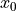to a final state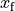in time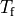we solve a point-to-point trajectory generation problem. We also set the initial and final inputs, which sets the vehicle velocity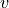and steering wheel angle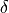at the endpoints.

# Define the endpoints of the trajectory
x0 = [0., -2., 0.]; u0 = [10., 0.]
xf = [100., 2., 0.]; uf = [10., 0.]
Tf = 10

# Define a set of basis functions to use for the trajectories
poly = fs.PolyFamily(6)

# Find a trajectory between the initial condition and the final condition
traj = fs.point_to_point(vehicle_flat, Tf, x0, u0, xf, uf, basis=poly)

# Create the trajectory
t = np.linspace(0, Tf, 100)
x, u = traj.eval(t)


Alternatively, we can solve an optimal control problem in which we minimize a cost function along the trajectory as well as a terminal cost:

# Define the cost along the trajectory: penalize steering angle
vehicle_flat, None, np.diag([0.1, 10]), u0=uf)

# Define the terminal cost: penalize distance from the end point
vehicle_flat, np.diag([1e3, 1e3, 1e3]), None, x0=xf)

# Use a straight line as the initial guess
timepts = np.linspace(0, Tf, 10)
initial_guess = np.array(
[x0[i] + (xf[i] - x0[i]) * timepts/Tf for i in (0, 1)])

# Solve the optimal control problem, evaluating cost at timepts
bspline = fs.BSplineFamily([0, Tf/2, Tf], 4)
traj = fs.solve_flat_ocp(
vehicle_flat, timepts, x0, u0, traj_cost,
terminal_cost=term_cost, initial_guess=initial_guess, basis=bspline)

x, u = traj.eval(t)


## Module classes and functions¶

 Base class for implementing basis functions for flat systems. BezierFamily(N[, T]) Bezier curve basis functions. BSplineFamily(breakpoints, degree[, ...]) B-spline basis functions. FlatSystem(forward, reverse[, updfcn, ...]) Base class for representing a differentially flat system. LinearFlatSystem(linsys[, inputs, outputs, ...]) Base class for a linear, differentially flat system. PolyFamily(N[, T]) Polynomial basis functions. SystemTrajectory(sys, basis[, coeffs, ...]) Class representing a trajectory for a flat system.
 point_to_point(sys, timepts[, x0, u0, xf, ...]) Compute trajectory between an initial and final conditions. solve_flat_ocp`(sys, timepts[, x0, u0, ...]) Compute trajectory between an initial and final conditions.